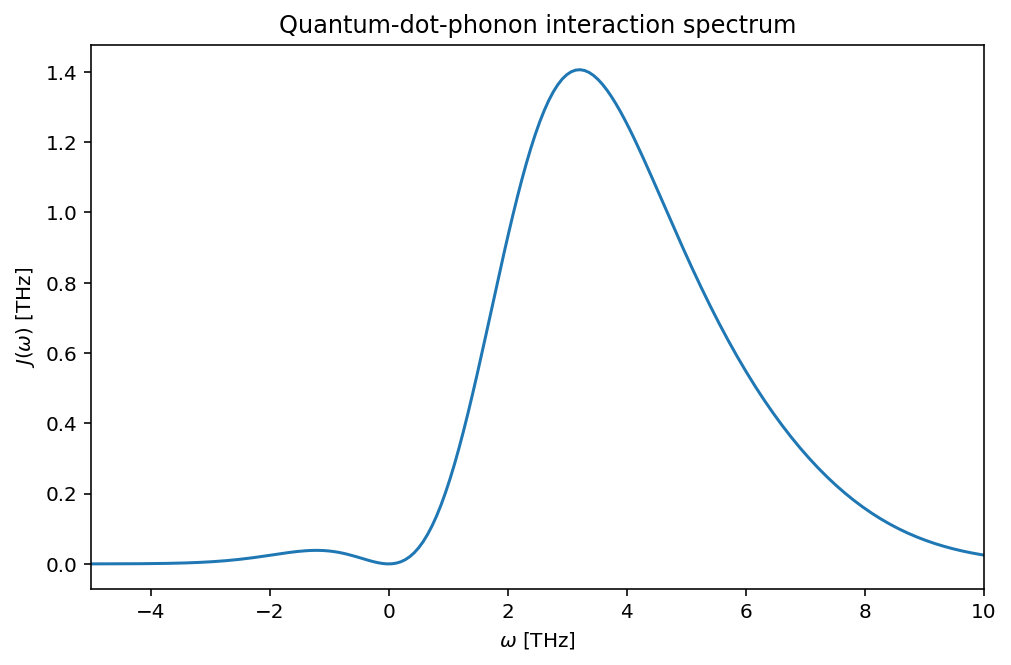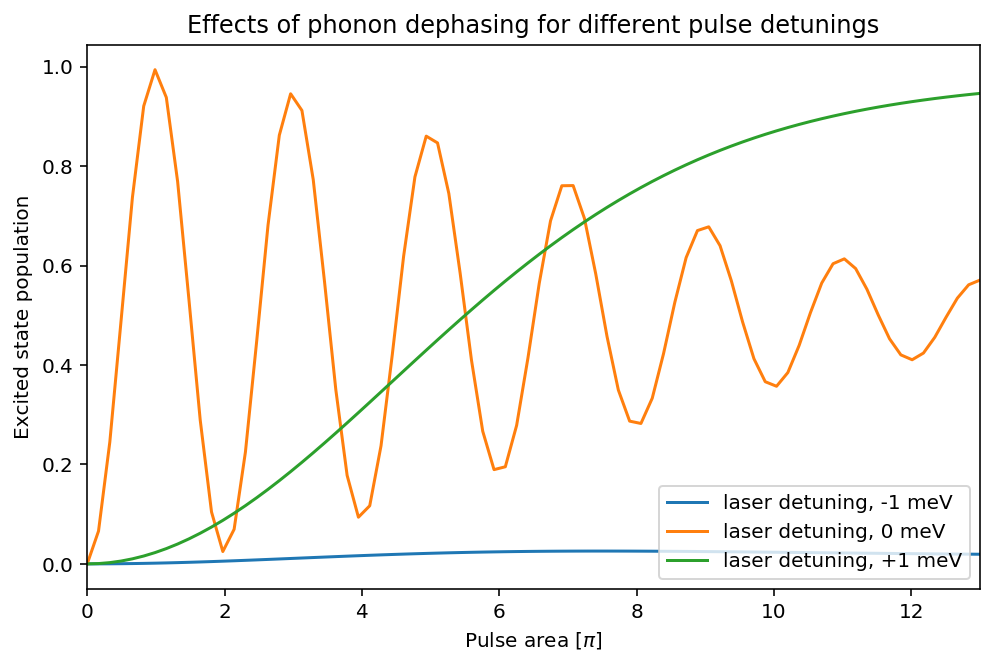# QuTiP example: Phonon-assisted initialization using the time-dependent Bloch-Redfield master equation solver¶

K.A. Fischer, Stanford University

This Jupyter notebook demonstrates how to use the time-dependent Bloch-Redfield master equation solver to simulate the phonon-assited initialization of a quantum dot, using QuTiP: The Quantum Toolbox in Python. The purpose is to show how environmentally-driven dissipative interactions can be leveraged to initialize a quantum dot into its excited state. This notebook closely follows the work, Dissipative preparation of the exciton and biexciton in self-assembled quantum dots on picosecond time scales, Phys. Rev. B 90, 241404(R) (2014).

In :
%matplotlib inline
%config InlineBackend.figure_format = 'retina'

In :
import matplotlib.pyplot as plt
import numpy as np
import itertools

In :
from qutip import *
from numpy import *


## Introduction¶

The quantum two-level system (TLS) is the simplest possible model to describe the quantum light-matter interaction between light and an artificial atom (quantum dot). While the version in the paper (both experiment and simulation) used a three-level system model, I decided to show only a TLS model here to minimize the notebook's runtime.

In the version we simulate here, the system is driven by a continuous-mode coherent state, whose dipolar interaction with the system is represented by the following Hamiltonain

$$H =\hbar \omega_0 \sigma^\dagger \sigma + \frac{\hbar\Omega(t)}{2}\left( \sigma\textrm{e}^{-i\omega_dt} + \sigma^\dagger \textrm{e}^{i\omega_dt}\right),$$

where $\omega_0$ is the system's transition frequency, $\sigma$ is the system's atomic lowering operator, $\omega_d$ is the coherent state's center frequency, and $\Omega(t)$ is the coherent state's driving strength.

The time-dependence can be removed to simplify the simulation by a rotating frame transformation. Then,

$$H_r =\hbar \left(\omega_0-\omega_d\right) \sigma^\dagger \sigma + \frac{\hbar\Omega(t)}{2}\left( \sigma+ \sigma^\dagger \right).$$

Additionally, the quantum dot exists in a solid-state matrix, where environmental interactions are extremely important. In particular, the coupling between acoustic phonons and the artifical atom leads to important dephasing effects. While the collapse operators of the quantum-optical master equation can be used to phenomenologically model these effects, they do not necessarily provide any direct connection to the underlying physics of the system-environmental interaction.

Instead, the Bloch-Redfield master equation allows for a direct connection between the quantum dynamics and the underlying physical interaction mechanism. Furthermore, the interaction strengths can be derived from first principles and can include complex power-dependences, such as those that exist in the quantum-dot-phonon interaction. Though we note that there are important non-Markovian effects which are now being investigated, e.g. in the paper Limits to coherent scattering and photon coalescence from solid-state quantum emitters, Phys. Rev. B 95, 201305(R) (2017).

### Problem parameters¶

Note, we use units where $\hbar=1$.

In :
n_Pi = 13                                     # 8 pi pulse area

Om_list = np.linspace(0.001, n_Pi, 80)        # driving strengths
wd_list_e = np.array([-1, 0, 1])            # laser offsets in meV
wd_list = wd_list_e*1.5                       # in angular frequency
tlist = np.linspace(0, 50, 40)                # tmax ~ 2x FWHM

# normalized Gaussian pulse shape, ~10ps long in energy
t0 = 17 / (2 * np.sqrt(2 * np.log(2)))
#pulse_shape = np.exp(-(tlist - 24) ** 2 / (2 * t0 ** 2))

pulse_shape = '0.0867 * exp(-(t - 24) ** 2 / (2 * {0} ** 2))'.format(t0)


### Setup the operators, Hamiltonian, and initial state¶

In :
# initial state
psi0 = fock(2, 1)      # ground state

# system's atomic lowering operator
sm = sigmam()

# Hamiltonian components
H_S = -sm.dag() * sm   # self-energy, varies with drive frequency
H_I = sm + sm.dag()

# we ignore spontaneous emission since the pulse is much faster than
# the decay time
c_ops = []


Below, we define the terms specific to the Bloch-Redfield solver's system-environmental coupling. The quantum dot couples to acoustic phonons in its solid-state environment through a dispersive electron-phonon interaction of the form

$$H_\textrm{phonon}=\hbar J(\omega)\sigma^\dagger \sigma,$$

where $J(\omega)$ is the spectra density of the coupling.

In :
# operator that couples the quantum dot to acoustic phonons
a_op = sm.dag()*sm

# This spectrum is a displaced gaussian multiplied by w^3, which
# models coupling to LA phonons. The electron and hole
# interactions contribute constructively.

"""
# fitting parameters ae/ah
ah = 1.9e-9            # m
ae = 3.5e-9            # m
# GaAs material parameters
De = 7
Dh = -3.5
v = 5110               # m/s
rho_m = 5370           # kg/m^3
hbar = 1.05457173e-34  # Js
T = 4.2                # Kelvin, temperature

# results in ~3THz angular frequency width, w in THz
# zero T spectrum, for w>0
J = 1.6*1e-13*w**3/(4*numpy.pi**2*rho_m*hbar*v**5) * \
(De*numpy.exp(-(w*1e12*ae/(2*v))**2) -
Dh*numpy.exp(-(w*1e12*ah/(2*v))**2))**2

# for temperature dependence, the 'negative' frequency
# components correspond to absorption vs emission

# w > 0:
JT_p = J*(1 + numpy.exp(-w*0.6582119/(T*0.086173)) / \
(1-numpy.exp(-w*0.6582119/(T*0.086173))))
# w < 0:
JT_m = -J*numpy.exp(w*0.6582119/(T*0.086173)) / \
(1-numpy.exp(w*0.6582119/(T*0.086173)))
"""

# the Bloch-Redfield solver requires the spectra to be
# formatted as a string
spectra_cb =' 1.6*1e-13*w**3/(4*pi**2*5370*1.05457173e-34*5110**5) * ' + \
'(7*exp(-(w*1e12*3.5e-9/(2*5110))**2) +' + \
'3.5*exp(-(w*1e12*1.9e-9 /(2*5110))**2))**2 *' + \
'((1 + exp(-w*0.6582119/(4.2*0.086173)) /' + \
'(1+1e-9-exp(-w*0.6582119/(4.2*0.086173))))*(w>=0)' + \
'-exp(w*0.6582119/(4.2*0.086173)) /' + \
'(1+1e-9-exp(w*0.6582119/(4.2*0.086173)))*(w<0))'


## Visualize the dot-phonon interaction spectrum¶

$J(\omega)$ has two components that give rise to its shape: a rising component due to the increasing acoustic phonon density of states and a roll-off that occurs due to the physical size of the quantum dot.

In :
spec_list = np.linspace(-5, 10, 200)

plt.figure(figsize=(8, 5))
plt.plot(spec_list, [eval(spectra_cb.replace('w', str(_))) for _ in spec_list])
plt.xlim(-5, 10)
plt.xlabel('$\omega$ [THz]')
plt.ylabel('$J(\omega)$ [THz]')
plt.title('Quantum-dot-phonon interaction spectrum');## Calculate the pulse-system interaction dynamics¶

The Bloch-Redfield master equation solver takes the Hamiltonian time-dependence in list-string format. We calculate the final population at the end of the interaction of the pulse with the system, which represents the population initialized into the excited state.

In :
# we will calculate the dot population expectation value
e_ops = [sm.dag()*sm]

# define callback for parallelization
def brme_step(args):
wd = args
Om = args
H = [wd * H_S, [Om * H_I, pulse_shape]]

# calculate the population after the pulse interaction has
# finished using the Bloch-Redfield time-dependent solver
return qutip.brmesolve(H, psi0, tlist, [[a_op, spectra_cb]],
e_ops,options=Options(rhs_reuse=True)).expect[-1]

# use QuTiP's builtin parallelized for loop, parfor
results = parfor(brme_step, itertools.product(wd_list, Om_list))

# unwrap the results into a 2d array
inv_mat_X = np.array(results).reshape((len(wd_list), len(Om_list)))


### Visualize the quantum dot's initialization fidelity¶

Below, consider the trace of excited state occupation for increasing pulse area at a detuning of $\omega_d-\omega_L=0$. Here, the oscillations represent the standard Rabi oscillations of a driven two-level system, damped for increasing pulse area by a Markovian-like dephasing. This damping could be represented with a power-dependent collapse operator in the normal quantum-optical master equation. However, for nonzero pulse detunings, the results are quite nontrivial and difficult to model with a collapse operator. Herein lies the power of the Bloch-Redfield approach: it captures the dephasing in a more natural basis, the dressed atom basis, from first principles.

In this basis, the dispersive phonon-induced dephasing drives a population difference between the dressed states. This amounts to driving the system towards a dissipative quasi-steady state that initializes the population into the excited state with almost unity fidelity. The initialization effect is very insensitive to precise pulse area or laser detuning, as discussed in our paper, and hence is a powerfully robust way to pump a quantum dot into its excited state. Below is an example trace showing this dissipative initialization for a +1meV laser detuning. The high fidelity of the initialization relies on a low temperature bath that prefers phonon emission over absorption. As a complement, for a laser detuning of -1meV, the excited state is barely populated.

In :
plt.figure(figsize=(8, 5))

plt.plot(Om_list, inv_mat_X)
plt.plot(Om_list, inv_mat_X)
plt.plot(Om_list, inv_mat_X)

plt.legend(['laser detuning, -1 meV',
'laser detuning, 0 meV',
'laser detuning, +1 meV'], loc=4)

plt.xlim(0, 13)
plt.xlabel('Pulse area [$\pi$]')
plt.ylabel('Excited state population')
plt.title('Effects of phonon dephasing for different pulse detunings');## Versions¶

In :
from qutip.ipynbtools import version_table

version_table()

Out:
SoftwareVersion
QuTiP4.3.0.dev0+aca609e9
Numpy1.13.1
SciPy0.19.1
matplotlib2.0.2
Cython0.26
Number of CPUs2
BLAS InfoINTEL MKL
IPython6.1.0
Python3.6.2 |Anaconda custom (x86_64)| (default, Jul 20 2017, 13:14:59) [GCC 4.2.1 Compatible Apple LLVM 6.0 (clang-600.0.57)]
OSposix [darwin]
Wed Aug 23 22:40:21 2017 MDT